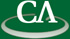Polynomials, permutations, prime ideals, and factoring polynomials modulo p.

Michael Rosen.

Friday July 7th at 3:00pm in K9509.

```Abstract:

The talk began as an attempt to answer the following question - if f(x) is an
irreducible polynomial with integer coefficients, does it remain irreducible
modulo p for infinitely many primes p? It turns out that the answer is sometimes
yes and sometimes no.  One can give the answer satisfactorily and even give the
Dirichlet density of the set of p for which the reduction is irreducible.

```